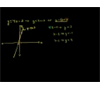## Want to see ALL questions on this topic?

### Upgrade to PLUS+ for €35 to see all past questions## You need to have an account to continue

### Algebra - Inequalities Videos & Notes

All
Videos
Websites
Notes
5 Videos & Notes
•How to Solve Inequalities (NancyPi)
MIT grad explains solving inequalities. This video focuses on solving linear inequalities. It shows when to switch the sign of the inequality, if you divide or multiply by a negative number, and is an introduction to how to solve inequalities in algebra. To skip ahead: 1) For a basic example of SOLVING AN INEQUALITY, skip to time 00:34. 2) For when you have to FLIP THE INEQUALITY SIGN when DIVIDING BY A NEGATIVE number, skip to 1:26. 3) For when you need to reverse the inequality symbol because you're MULTIPLYING BY A NEGATIVE number, skip to 2:42. The problems here are multi-step inequalities, or two-step inequalities. Nancy formerly of MathBFF explains the steps. Follow Nancy on Instagram: https://instagram.com/nancypi Twitter: https://twitter.com/nancypi Algebraic equations and inequalities: what is inequality math? What does inequality mean? An inequality is a number sentence that uses an inequality symbol instead of an equality symbol (=), which means it has a greater than sign, less than sign, greater than or equal to sign, or less than or equal to sign, instead of the normal equal sign in an equation. One linear inequality definition is: an inequality that involves a linear algebraic expression and inequality signs. How to find the solution of an inequality algebra problem: 1) A simple, BASIC LINEAR INEQUALITY example you might see on a solving inequalities worksheet or problem set is the expression 2x + 3 [less than or equal to] 11. You can solve for x just like you would for an equality, or normal equation, by trying to get x alone. This is an example of two-step inequalities, or multi-step inequalities. The first step is to subtract the constant 3 from both sides, in order to move it to the right hand side, so that you have 2x [less than or equal to] 8. The second step is to get x alone on the left side by dividing both sides by 2 so that your answer for the inequality is x [less than or equal to] 4. In this case, solving the inequality used the exact same steps you would use to solve an "equal sign" equation. Sometimes the steps are different from solving normal math equations. Solving for x is the same as solving a linear equality (-2x + 3 = 11) except that if you ever have to divide by a negative number or multiply by a negative number while solving for x, you must flip the sign, or switch the direction of your inequality symbol less than becomes greater than, for example). Here are the two cases of inequality problems WHEN YOU NEED TO REVERSE THE SIGN of the inequality because you're multiplying or dividing both sides of the inequality by a negative number: 2) DIVIDING EXAMPLE: For an inequality like -2x + 3 [less than or equal to] 11, after moving the constant 3 to the right side, you will need to divide both sides by negative 2 in order to get x alone on the left side for the solution. WHEN YOU DIVIDE BY A NEGATIVE NUMBER, you need to reverse the direction of the inequality symbol in your expression. In this example, after you divide out -2, you get x [greater than or equal to] -4 (instead of x less than or equal to -4) for the final solution. This example was also a two-step inequality problem. 3) MULTIPLYING EXAMPLE: For examples like (-1/2)x + 4 [greater than] 7, when you've moved the constant to the right side and have the inequality (-1/2)x [greater than] 3, the fastest way to get x alone is to clear the fraction by multiplying both sides by -2. This will cancel the 2 in the denominator and remove the negative sign in front of x on the left side. But WHEN YOU MULTIPLY BY A NEGATIVE, you have to flip the direction of the inequality symbol so that your answer is x [less than] -6 instead of x [greater than] -6. This problem was also a multi-step inequality. Note on inequality rules: you don't have to switch the symbol if you're adding a negative number or subtracting a negative number: switching the inequality sign is only for when you're multiplying or dividing by a negative number. For quadratic inequalities examples, compound inequalities, inequality math, inequality algebra, inequality equations, inequality symbols, inequality notation, and more algebra solver, equation solver, and inequality calculator practice problems that come up on worksheets, as well as more algebra, algebra 2, and college algebra mathematics videos, check out: http://nancypi.com
read more
•Solving and Graphing Linear Inequalities
Solving and graphing linear inequalities in two variables. More free lessons at: http://www.khanacademy.org/video?v=eF6zYNzlZKQ Note: All Khan Academy content is available for free at (www.khanacademy.org)
read more
•Graphing Inequalities
How to: Graph linear inequalities. More free lessons at: http://www.khanacademy.org/video?v=eF6zYNzlZKQ Note: All Khan Academy content is available for free at (www.khanacademy.org)
read more